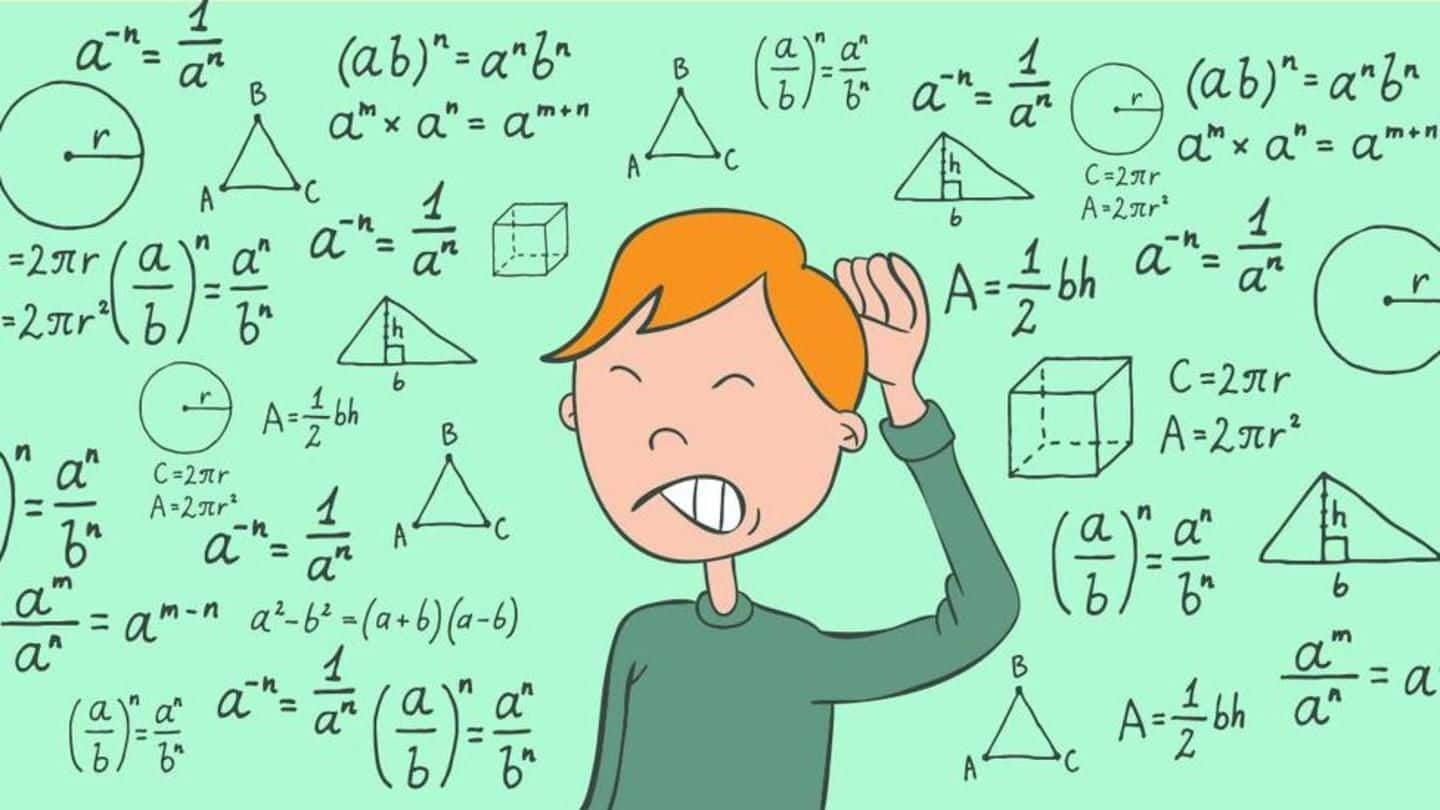# 110+ Topic-Wise Maths Tricks- Fast Calculation Tricks

##110+ Topic-Wise Maths Tricks- Fast Calculation Tricks:- Quantitative Aptitude or Numerical Ability section does not need any introduction. You know it forms a significant part of almost every Bank/Insurance/SSC/Railways/Government exam. Lakhs of candidates appear in this exams every year. To get your desired job in this pool of competition, your approach should be unique and different.

### SHORTCUT TRICK -1 (Distance Calculation Between 2 points)

To calculate the distance between 2 points when an object is traveling to and fro from one point to another, and just the speed and time taken is given, you can use the below formula directly to calculate the distance.

d= (t1+t2)((a+b)/ab)

Some other useful articles:-

• ### 1000 One liner Lucent gk questions in Hindis

Q1) If a man takes 3 hrs and 5 hrs respectively in going from point A to B then from B to A at 6km/hr and 8 km/hr respectively. What will be the distance between points A & B?

Ans: Directly apply the formula to get the answer: d= (t1+t2)((a+b)/ab)

d= (3+5)((6+8)/48) = 8*(14/48) = 14/6 km.

Where T1 & T2 are the time taken, a & b are the respective speeds and d = distance.

## Download Maths Tricks PDF Part-I

#### SHORTCUT TRICK -2 (Multiplication By 11)

If a number is given to be multiplied by 11, you can use the following method to calculate the answer. Suppose you have 79*11. Put the number of 79 at units and 100’s place and put the total of that number in between. if the total exceeds 10 add 1 the digit at 100’s place thus bringing your answer.

ab*11 = a(a+b)b,

If a+b>10 add 1 to ‘a’ and keep the rest term intact. below is the sample question.

## 110+ Topic-Wise Maths Tricks- Fast Calculation Tricks

Q2) Calculate what is 79*11.

Ans: If you go by the regular formula you might not be able to do it verbally. This trick is applicable only when a number is said to be multiplied by ’11’ Here is a direct trick to solve this multiplication.

79*11 = 7() 9 = 7(16)9 = 869

### SHORTCUT TRICK – 3 (Calculation of Multiple Interest on CI)

If a loan is taken for more than 1 year and the rate of interest in varying for different years, you can directly use the formula given here to calculate the answer.

Amount = P[1+R1/100] [1+R2/100] [1+R3/100] where R1 = rate of interest for 1st year, R2 for the second year and so on.

### 110+ Topic-Wise Maths Tricks- Fast Calculation Tricks

Q3) If 40000 is given as loan for a period of 3 years with the interest rates 6%, 8% and 9% for the 1st, 2nd and 3rd year respectively. What is the total amount that has to be paid at the end of 3 years?

Ans: By going with the regular method you will have to calculate the CI for each year and then add the CI of next year to the previous years’ number.

Here is a direct trick to avoid this:

Amount = P[1+6/100] [1+8/100] [1+9/100] where R1 = rate of interest for 1st year(6%), R2 (8%) for the second year and so on. Put the values and get the answer. Repeat it for any other similar case.

### Download Maths Tricks PDF Part-II

Visit our Study Material Section for more free

Must Read DisclaimerGrabNaukri.com is only for the Educational Purpose & Govt. Job links provider. We are not owner of any Book, Notes, Magazine, PDF Materials, eBooks Available on it, neither it been Created nor Scanned. We are only provide the link and Material already Available on the Internet. If any violates the Law or there is a Problem so Please Just CONTACT US.